# Distribution realizations¶

The objective is to transform a random realization of the Uniform(0,1) distribution onto a random realization of a distribution which cumulative density function is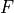.

Several classical techniques exist:

• The inversion of the CDF: if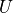is distributed according to the uniform distribution over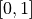(the bounds 0 and 1 may or may not be included), then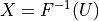is distributed according to the CDF. If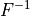has a simple analytical expression, it provides an efficient way to generate realizations of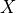. Two points need to be mentioned:

1. If the expression of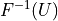involves the quantity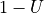instead of, it can be replaced byasandare identically distributed;

2. The numerical range ofis always bounded (i.e. the interval over which it is invertible) even if its mathematical range is unbounded, and the numerical range may not preserve the symmetry of its mathematical counterpart. It can lead to biased nonuniform generators, even if this bias is usually small. For example, using standard double precision, the CDF of the standard normal distribution is numerically invertible over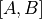with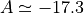and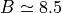.

• The rejection method: suppose that one want to sample a random variablewith a continuous distribution with PDF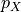and that we know how to sample a random variable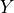with a continuous distribution with PDF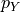. We suppose that there exist a positive scalar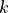such that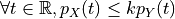. The rejection method consists of the following steps:

1. Generate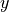according to;

2. Generate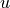according to a random variableindependent ofand uniformly distributed over;

3. If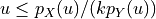, acceptas a realization of, else return to step 1.

The rejection method can be improved in several ways:

• If the evaluation of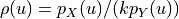is costly, and if one knows a cheap function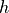such that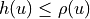, then one can first check if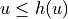and directly acceptif the test is positive (quick acceptance step). This is very effective if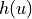can be evaluated from quantities that have to be computed to evaluate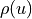:is a kind of cheap version of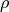. The same trick can be use if one knows a cheap function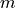such that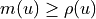: one checks if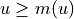and directly rejectif the test is positive (quick rejection test). The combination of quick acceptation and quick rejection is called a squeeze.

• The test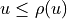can be replaced by an equivalent one but much more computationally efficient.

• The transformation method: suppose that one want to sample a random variable that is the image by a simple transformation of another random variable (or random vector) that can easily be sampled. It is easy to sample this last random variable (or vector) and then transform this realization through the transformation to get the needed realization. This method can be combined with the rejection method for example, to buildorimplicitly.

• The sequential search method (discrete distributions): it is a particular version of the CDF inversion method, dedicated to discrete random variables. One generates a realizationof a random variableuniformly distributed over, then we search the smallest integersuch that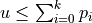, where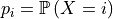.

• The stable distribution method (Archimedean copulas): to be detailed.

• The conditional CDF inversion (general copula or general multivariate distributions): this method is a general procedure to sample a multivariate distribution. One generate in sequence a realization of the first marginal distribution, then a realization of the distribution of the second component conditionally to the value taken by the first component, and so on. Each step is done by inversion of the conditional CDF of the componentwith respect to the value taken by the preceding components.

• The ratio of uniforms method: this is a special combination of the rejection method and the transformation method that has gained a great popularity due to its concision and its versatility. Let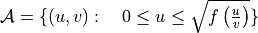. If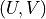is a random vector uniformly distributed over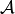, then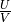has density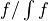. The generation ofis done by a rejection method, using a bounded enclosing region of. It can be done if and only if both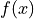and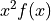are bounded. This method can be enhanced by using quick acceptance and quick rejection steps.

• The ziggurat method: this method allows for a very fast generation of positive random variate with decreasing PDF. The graph of the PDF is partitioned into horizontal slices of equal mass, the bottom slice covering the whole support of the PDF. All these slices have a maximal enclosed rectangle (the top one being empty) and a minimal enclosing rectangle (the bottom one not being defined). Then, one generate a discrete uniform random variable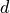over the number of slice. It selects a slice, and if this slice has both an enclosed and an enclosing rectangle, one generates a realization of a continuous uniform random variable on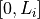,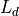being the length of the enclosing rectangle of slice. The enclosing and the enclosed rectangles define an efficient squeeze for a rejection method. If the bottom slice is selected, one has to sample the tail distribution conditional to the length of the enclosed rectangle: it is the only case where a costly non-uniform random number has to be computed. If the number of slices is large enough, this case appears only marginally, which is the main reason for the method efficiency.

The techniques implemented in each distribution are:

• Arcsine: CDF inversion

• Bernoulli: CDF inversion

• Beta:

• CDF inversion if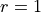or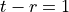.

• Rejection (Johnk’s method) for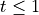.

• Rejection (Cheng’s method) for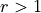,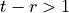.

• Rejection (Atkinson and Whittaker method 1) for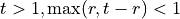.

• Rejection (Atkinson and Whittaker method 2) for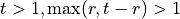.

• Binomial: Squeeze and Reject method: See [hormann1993].

• Burr: CDF inversion.

• Chi: Transformation.

• ChiSquare: See the Gamma distribution.

• ClaytonCopula: Conditional CDF inversion.

• ComposedCopula: Simulation of the copula one by one then association.

• ComposedDistribution: Simulation of the copula and the marginal with CDF inversion.

• Dirac: Return the supporting point.

• Dirichlet: Transformation.

• Epanechnikov: CDF inversion.

• Exponential: CDF inversion.

• Fisher-Snedecor: Transformation.

• FrankCopula: Conditional CDF inversion.

• Gamma: Transformation and rejection, see [marsaglia1993].

• Geometric: CDF inversion.

• Generalized Pareto: CDF inversion.

• GumbelCopula: Stable distribution.

• Gumbel: CDF inversion.

• Histogram: CDF inversion.

• IndependentCopula: Transformation.

• InverseNormal: Transformation.

• KernelMixture: Transformation.

• Kpermutaions: Knuth’s algorithm.

• Laplace: CDF inversion.

• Logistic: CDF inversion.

• LogNormal: Transformation.

• LogUniform: Transformation.

• Meixner: Uniform ratio method.

• MinCopula: Transformation.

• Mixture: Transformation.

• MultiNomial: Conditional CDF inversion.

• Non Central Chi Square: Transformation.

• NegativeBinomial: Conditional simulation (Poisson|Gamma)

• Non Central Student: Transformation.

• NormalCopula: Transformation of independent Normal realizations.

• Normal:

• 1D: Ziggurat method

• nD: Transformation of independent Normal realizations

• Poisson:

• Sequential search for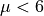• Ratio of uniforms for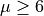• RandomMixture: Transformation

• Rayleigh: CDF inversion

• Rice: Transformation

• Skellam: Transformation

• SklarCopula: Conditional CDF inversion by Gaussian quadrature and numerical inversion

• Student: Transformation

• Trapezoidal: CDF inversion

• Triangular: CDF inversion

• TruncatedDistribution: on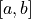we notethe CDF of the non truncated distribution

• if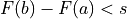: CDF inversion

• if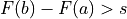: rejection

By default,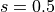(modifiable)

• TruncatedNormal:

• small truncation interval: CDF inversion

• large truncation interval: rejection

• Uniform: Transformation.

• UserDefined: Sequential search.

• WeibullMin: CDF inversion.

• Zipf-Mandelbrot: Bisection search.